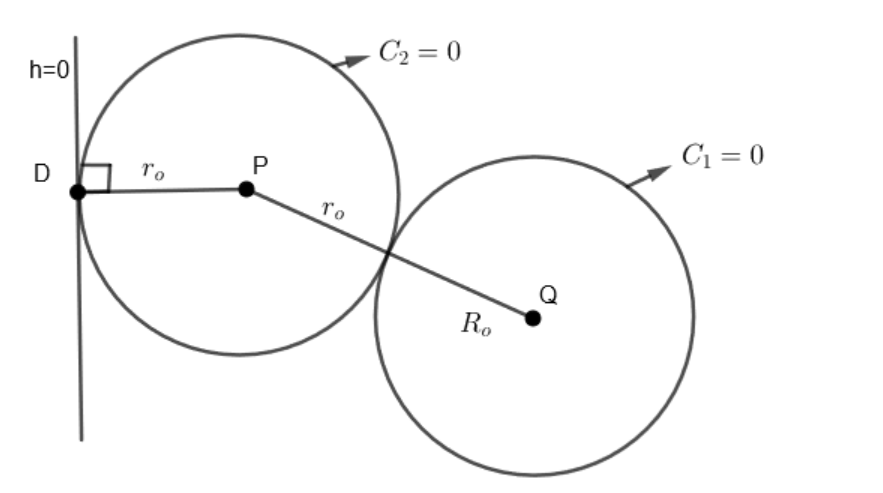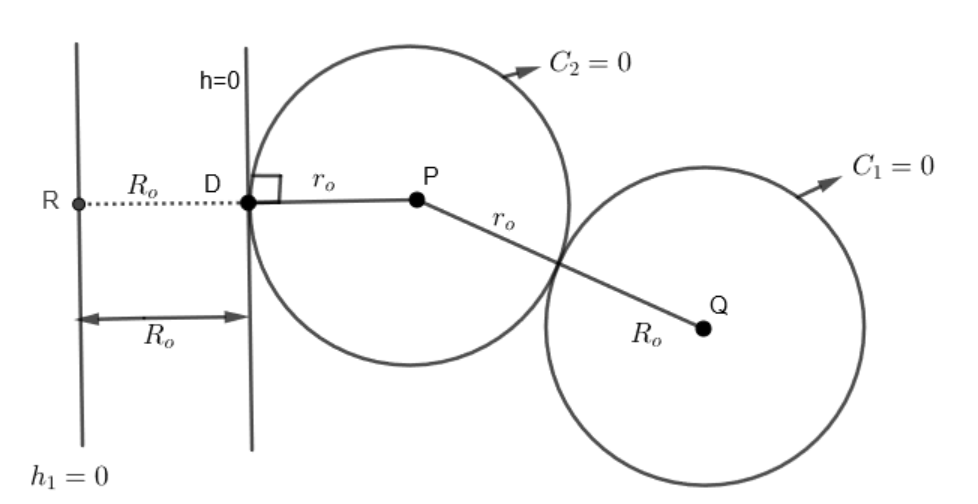Courses
Courses for Kids
Free study material
Free LIVE classes
MoreLIVE
Join Vedantu’s FREE Mastercalss

# If a circle be drawn, so as always to touch a given straight line and also a given circle, prove that the locus of its center is a parabola.Verified
365.7k+ views
Hint: Take the given condition on plane and draw a line parallel to the given line at the distance equal to radius of given circle.
Given: A circle and a straight line.
We have to prove that locus of center of circle drawn touching given circle and straight line is parabola.Complete step by step solution:
Let the given line be $h=0$ and given circle be ${{C}_{1}}=0$ with center $Q$ and radius ${{R}_{o}}$.
Now, circle is drawn touching line $h=0$ and circle ${{C}_{1}}=0$ is ${{C}_{2}}=0$ with center $P$ and radius ${{r}_{o}}$.
From diagram, we can see that,
$PQ={{r}_{o}}+{{R}_{o}}.....\left( i \right)$
As, ${{r}_{o}}$ and $PD$ are radius of circle ${{C}_{2}}$,
We get, ${{r}_{o}}=PD$
Therefore, $PQ=PD+{{R}_{o}}....\left( ii \right)$
Construct a line ${{h}_{1}}=0$ parallel to $h=0$ at a distance ${{R}_{o}}$ from $h=0$ and extend $PQ$ as shown.Since $h$ is parallel to ${{h}_{1}}$,
Therefore, $DR={{R}_{o}}...\left( iii \right)$
Now, we know that
$PQ={{r}_{o}}+{{R}_{o}}...\left( iv \right)$
Therefore, $PQ=PD+{{R}_{o}}$….from equation $\left( i \right)$
Also, $PR=PD+DR\text{ }\!\![\!\!\text { by diagram }\!\!]\!\!\text{ }$
Therefore, $PR={{r}_{o}}+{{R}_{o}}....\left( v \right)\left[ \text{From equation }\left( iii \right) \right]$
Now, we know that any point on parabola has equal distances from its directrix and focus.
Similarly, here point $P$ which is center of circle has equal distance $\left[ {{r}_{o}}+{{R}_{o}} \right]$ from ${{h}_{1}}=0$ and point $Q$.
Therefore, here ${{h}_{1}}$ is behaving as directrix and $Q$ as a focus of parabola.
Hence, locus of $P$ is parabola.

Note: Students must understand the physical significances of coordinate geometry and importance of theory, specifically parabola in the given question.

Last updated date: 28th Sep 2023
Total views: 365.7k
Views today: 11.65k